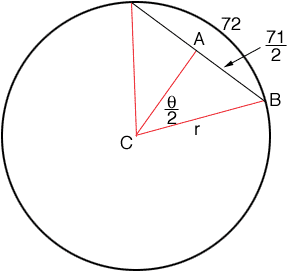Quandaries and Queries Robert Student Grade 9-10   Given only the length of an arc (72) & the length of its cord (71), how to find the radius? Hi Robert, Unfortunately there isn't a nice answer to your question. I drew a diagram and labeled various parts.A is the midpoint of the chord so |AB| = 71/2. I let theta be the angle at the center of the circle subtended by the arc. Theta is in radians. Triangle ACB is a right triangle so sin( theta/2) = 71/2r The length of the arc subtended by the angle theta is 72 so 72 = rtheta Thus theta = 72/r Hence, substituting into the first equation and simplifying I get  71/2 = rsin( 36/r) The best you can do now is use some numerical method to approximate r. Penny